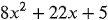sorenshalini13

Lv5

APJ Abdul Kalam Technological University

0 Followers
0 Following
0 Helped

Published100

Subjects

Answer: Radical - The √ symbol that is used to denote square root or nth roots...
Answer: Delta Symbol: Change Uppercase delta (Δ) at most times means “change” ...
Answer: A series can be represented in a compact form, called summation or sig...
Answer: In mathematics, a binary relation is a general concept that defines so...
Answer: Or simply, a bunch of points (ordered pairs). In other words, the rela...
Answer: In mathematics, a (real) interval is a set of real numbers that contai...
Answer: Estimation of a number is a reasonable guess of the actual value to ma...
Answer: Rationalization can be considered as the process used to eliminate a r...
Answer: 79/60Step-by-step explanation:= 11/12 + 2/5 = (55+24)/60 = 79/60
Answer: 5/2Step-by-step explanation:= 2.5 = 25/10 = 5/2
Answer: 5/2Step-by-step explanation:= 2.5 = 25/10 = 5/2
Answer: 5/2Step-by-step explanation:= 2.5 = 25/10 = 5/2
Answer: 5/2Step-by-step explanation:= 2.5 = 25/10 = 5/2
Answer: 3/16Step-by-step explanation: = 0.1875 = 1875/10000 = 375/2000 = 75/40...
Answer: 13/10Step-by-step explanation:= 1.3 = 13/10Answer: x= (-5/2, -1/4)Step-by-step explanation:= 8x^(2)+22x+5 = 8x^(2)+ 2x+ 2...
11. I _____ in school almost all day. (past tense of be)
12. My sister _____ money for college. (future tense of save)
13. After weeks of looking, Jim _____ a job. (past tense of find)
14. She _____ the office about the meeting. (future tense of notify)
15. _____ you please _____ the door for me? (future tense of open)
16. The twins _____ almost always together. (present tense of be)
17. They _____ for three days to get here. (past tense of drive
18. I _____ my new radio with me to the beach. (past tense of bring)
19. Consuelo _____ for the job tomorrow. (future tense of interview)
20. It _____  almost time to leave for class (future tense of be)
21. I _____ never _____  his face. (future tense of forget)
22. Jacob  _____ his jacket on a nail. (past tense of hang)
23. I think I _____  my car keys in the grass. (past tense of lose)
24. The French club _____ a debate tomorrow. (future tense of hold)
25. Our side _____  the mock trial. (past tense of win
26. Who  _____ the Declaration of Independence first? (past tense of sign)
27. I think he _____ about the surprise party. (present tense of know)
28. Alfonso _____ us paint the room. (future tense of help)
29. Shakespeare many fine plays. (past tense of write)
30. Last week Beth _____ ten laps. (past tense of swim)
31. The gym _____ soon _____ new equipment. (future tense of need)
32. Arturo _____ almost all the potato salad. (past tense of eat)
33. I _____ my paycheck to the bank. (future tense of take)
34. His old car  _____ down on the highway. (past tense of break)
35. The crowd of people  _____ past us. (past tense of run)
36. Mom  _____ about the boys. (present tense of worry)
37. My quarter  _____ in the parking meter. (past tense of jam
38. The conductor _____ us the right stop. (future tense of tell)
39. Grandma  _____ how to knit. (past tense of teach)
40. The batter  _____ a swing at the ball.(past tense of take)
Answer: Step-by-step explanation:11. I _was____ in school almost all day. (pas...
Answer: 3/50Step-by-step explanation:= 6% = 6/100 = 3/50
Answer: 5/2Step-by-step explanation:= 2.5 = 25/10 = 5/2